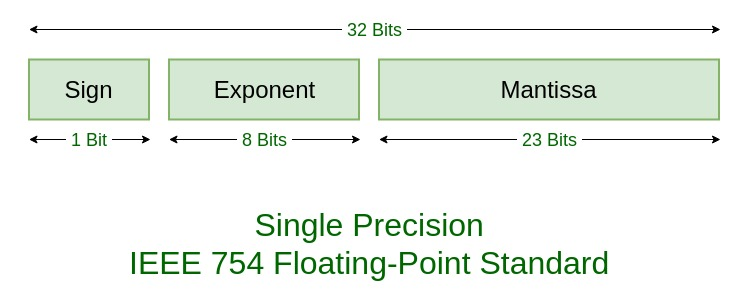GFG App
Open AppBrowser
Continue

# Python program to represent floating number as hexadecimal by IEEE 754 standard

Prerequisite : IEEE Standard 754 Floating Point Numbers
Given a floating point number, the task is to find the hexadecimal representation for the number by IEEE 754 standard.
The IEEE Standard for Floating-Point Arithmetic (IEEE 754) is a technical standard for floating-point computation which was established in 1985 by the Institute of Electrical and Electronics Engineers (IEEE). The standard addressed many problems found in the diverse floating point implementations that made them difficult to use reliably and reduced their portability. IEEE Standard 754 floating point is the most common representation today for real numbers on computers, including Intel-based PC’s, Macs, and most Unix platforms.Examples :

```Input :  -6744.90
Output :  C5D2C733

Input :  -263.3
Output :  C383A666```

Approach :

• Check whether the number is positive or negative. Save the sign as 0 for positive and 1 for negative, and then convert the number into positive if it is negative.
• Convert the floating point number to binary.
• Separate the decimal part and the whole number part.
• Calculate the exponent(E) and convert it to binary.
• Find the mantissa.
• Concatenate the sign of mantissa, exponent and the mantissa.

Let’s write a Python program to represent a floating number as hexadecimal by IEEE 754 standard.

## Python3

 `# Function for converting decimal to binary` `def` `float_bin(my_number, places ``=` `3``): ` `    ``my_whole, my_dec ``=` `str``(my_number).split(``"."``)` `    ``my_whole ``=` `int``(my_whole)` `    ``res ``=` `(``str``(``bin``(my_whole))``+``"."``).replace(``'0b'``,'')`   `    ``for` `x ``in` `range``(places):` `        ``my_dec ``=` `str``(``'0.'``)``+``str``(my_dec)` `        ``temp ``=` `'%1.20f'` `%``(``float``(my_dec)``*``2``)` `        ``my_whole, my_dec ``=` `temp.split(``"."``)` `        ``res ``+``=` `my_whole` `    ``return` `res`       `def` `IEEE754(n) : ` `    ``# identifying whether the number` `    ``# is positive or negative` `    ``sign ``=` `0` `    ``if` `n < ``0` `: ` `        ``sign ``=` `1` `        ``n ``=` `n ``*` `(``-``1``) ` `    ``p ``=` `30` `    ``# convert float to binary` `    ``dec ``=` `float_bin (n, places ``=` `p)`   `    ``dotPlace ``=` `dec.find(``'.'``)` `    ``onePlace ``=` `dec.find(``'1'``)` `    ``# finding the mantissa` `    ``if` `onePlace > dotPlace:` `        ``dec ``=` `dec.replace(``"."``,"")` `        ``onePlace ``-``=` `1` `        ``dotPlace ``-``=` `1` `    ``elif` `onePlace < dotPlace:` `        ``dec ``=` `dec.replace(``"."``,"")` `        ``dotPlace ``-``=` `1` `    ``mantissa ``=` `dec[onePlace``+``1``:]`   `    ``# calculating the exponent(E)` `    ``exponent ``=` `dotPlace ``-` `onePlace` `    ``exponent_bits ``=` `exponent ``+` `127`   `    ``# converting the exponent from` `    ``# decimal to binary` `    ``exponent_bits ``=` `bin``(exponent_bits).replace(``"0b"``,'') `   `    ``mantissa ``=` `mantissa[``0``:``23``]`   `    ``# the IEEE754 notation in binary     ` `    ``final ``=` `str``(sign) ``+` `exponent_bits.zfill(``8``) ``+` `mantissa`   `    ``# convert the binary to hexadecimal ` `    ``hstr ``=` `'0x%0*X'` `%``((``len``(final) ``+` `3``) ``/``/` `4``, ``int``(final, ``2``)) ` `    ``return` `(hstr, final)`   `# Driver Code` `if` `__name__ ``=``=` `"__main__"` `:` `    ``print` `(IEEE754(``263.3``))` `    ``print` `(IEEE754(``-``263.3``))`

Output:

```4383A666
C383A666```

Time complexity: The time complexity of this function is O(p) where p is the number of places of the decimal value passed to the function. This is because the function uses a for loop that runs for p iterations to convert the decimal value to binary.

Space complexity: The space complexity of this function is O(1), as the function uses only a few variables that are independent of the input size. The function does not use any data structures with size dependent on the input.

My Personal Notes arrow_drop_up